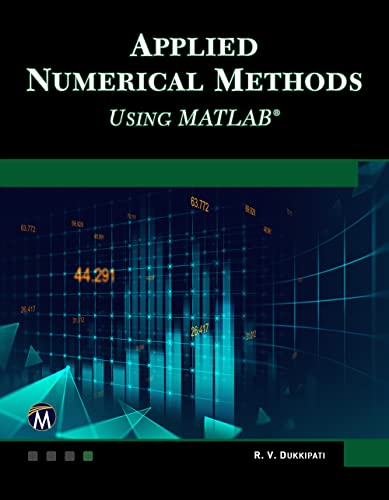# Applied Numerical Methods Using MATLAB

• Length: 950 pages
• Edition: 1
• Publisher:
• Publication Date: 2023-03-30
• ISBN-10: 1683928687
• ISBN-13: 9781683928683
Description

The book is designed to cover all major aspects of applied numerical methods, including numerical computations, solution of algebraic and transcendental equations, finite differences and interpolation, curve fitting, correlation and regression, numerical differentiation and integration, matrices and linear system of equations, numerical solution of ordinary differential equations, and numerical solution of partial differential equations. MATLAB is incorporated throughout the text and most of the problems are executed in MATLAB code. It uses a numerical problem-solving orientation with numerous examples, figures, and end of chapter exercises. Presentations are limited to very basic topics to serve as an introduction to more advanced topics.

FEATURES:

• Integrates MATLAB throughout the text
• Includes over 600 fully-solved problems with step-by-step solutions
• Limits presentations to basic concepts of solving numerical methods